# 10.1 Non-right triangles: law of sines  (Page 5/10)

 Page 5 / 10

## Verbal

Describe the altitude of a triangle.

The altitude extends from any vertex to the opposite side or to the line containing the opposite side at a 90° angle.

Compare right triangles and oblique triangles.

When can you use the Law of Sines to find a missing angle?

When the known values are the side opposite the missing angle and another side and its opposite angle.

In the Law of Sines, what is the relationship between the angle in the numerator and the side in the denominator?

What type of triangle results in an ambiguous case?

A triangle with two given sides and a non-included angle.

## Algebraic

For the following exercises, assume $\text{\hspace{0.17em}}\alpha \text{\hspace{0.17em}}$ is opposite side $\text{\hspace{0.17em}}a,\beta \text{\hspace{0.17em}}$ is opposite side $\text{\hspace{0.17em}}b,\text{\hspace{0.17em}}$ and $\text{\hspace{0.17em}}\gamma \text{\hspace{0.17em}}$ is opposite side $\text{\hspace{0.17em}}c.\text{\hspace{0.17em}}$ Solve each triangle, if possible. Round each answer to the nearest tenth.

$\alpha =43°,\gamma =69°,a=20$

$\alpha =35°,\gamma =73°,c=20$

$\alpha =60°,\text{\hspace{0.17em}}\text{\hspace{0.17em}}\beta =60°,\text{\hspace{0.17em}}\gamma =60°$

$a=4,\text{\hspace{0.17em}}\text{\hspace{0.17em}}\alpha =\text{\hspace{0.17em}}60°,\text{\hspace{0.17em}}\beta =100°$

$b=10,\text{\hspace{0.17em}}\beta =95°,\gamma =\text{\hspace{0.17em}}30°$

For the following exercises, use the Law of Sines to solve for the missing side for each oblique triangle. Round each answer to the nearest hundredth. Assume that angle $\text{\hspace{0.17em}}A\text{\hspace{0.17em}}$ is opposite side $\text{\hspace{0.17em}}a,\text{\hspace{0.17em}}$ angle $\text{\hspace{0.17em}}B\text{\hspace{0.17em}}$ is opposite side $\text{\hspace{0.17em}}b,\text{\hspace{0.17em}}$ and angle $\text{\hspace{0.17em}}C\text{\hspace{0.17em}}$ is opposite side $\text{\hspace{0.17em}}c.$

Find side $\text{\hspace{0.17em}}b\text{\hspace{0.17em}}$ when $\text{\hspace{0.17em}}A=37°,\text{\hspace{0.17em}}\text{\hspace{0.17em}}B=49°,\text{\hspace{0.17em}}c=5.$

$b\approx 3.78$

Find side $\text{\hspace{0.17em}}a$ when $\text{\hspace{0.17em}}A=132°,C=23°,b=10.$

Find side $\text{\hspace{0.17em}}c\text{\hspace{0.17em}}$ when $\text{\hspace{0.17em}}B=37°,C=21,\text{\hspace{0.17em}}b=23.$

$c\approx 13.70$

For the following exercises, assume $\text{\hspace{0.17em}}\alpha \text{\hspace{0.17em}}$ is opposite side $\text{\hspace{0.17em}}a,\beta \text{\hspace{0.17em}}$ is opposite side $\text{\hspace{0.17em}}b,\text{\hspace{0.17em}}$ and $\text{\hspace{0.17em}}\gamma \text{\hspace{0.17em}}$ is opposite side $\text{\hspace{0.17em}}c.\text{\hspace{0.17em}}$ Determine whether there is no triangle, one triangle, or two triangles. Then solve each triangle, if possible. Round each answer to the nearest tenth.

$\alpha =119°,a=14,b=26$

$\gamma =113°,b=10,c=32$

one triangle, $\text{\hspace{0.17em}}\alpha \approx 50.3°,\beta \approx 16.7°,a\approx 26.7$

$b=3.5,\text{\hspace{0.17em}}\text{\hspace{0.17em}}c=5.3,\text{\hspace{0.17em}}\text{\hspace{0.17em}}\gamma =\text{\hspace{0.17em}}80°$

$a=12,\text{\hspace{0.17em}}\text{\hspace{0.17em}}c=17,\text{\hspace{0.17em}}\text{\hspace{0.17em}}\alpha =\text{\hspace{0.17em}}35°$

two triangles, or

$a=20.5,\text{\hspace{0.17em}}\text{\hspace{0.17em}}b=35.0,\text{\hspace{0.17em}}\text{\hspace{0.17em}}\beta =25°$

$a=7,\text{\hspace{0.17em}}c=9,\text{\hspace{0.17em}}\text{\hspace{0.17em}}\alpha =\text{\hspace{0.17em}}43°$

two triangles, or

$a=7,b=3,\beta =24°$

$b=13,c=5,\gamma =\text{\hspace{0.17em}}10°$

two triangles, $\text{\hspace{0.17em}}\alpha \approx 143.2°,\beta \approx 26.8°,a\approx 17.3\text{\hspace{0.17em}}$ or $\text{\hspace{0.17em}}{\alpha }^{\prime }\approx 16.8°,{\beta }^{\prime }\approx 153.2°,{a}^{\prime }\approx 8.3$

$a=2.3,c=1.8,\gamma =28°$

$\beta =119°,b=8.2,a=11.3$

no triangle possible

For the following exercises, use the Law of Sines to solve, if possible, the missing side or angle for each triangle or triangles in the ambiguous case. Round each answer to the nearest tenth.

Find angle $A$ when $\text{\hspace{0.17em}}a=24,b=5,B=22°.$

Find angle $A$ when $\text{\hspace{0.17em}}a=13,b=6,B=20°.$

$A\approx 47.8°\text{\hspace{0.17em}}$ or $\text{\hspace{0.17em}}{A}^{\prime }\approx 132.2°$

Find angle $\text{\hspace{0.17em}}B\text{\hspace{0.17em}}$ when $\text{\hspace{0.17em}}A=12°,a=2,b=9.$

For the following exercises, find the area of the triangle with the given measurements. Round each answer to the nearest tenth.

$a=5,c=6,\beta =\text{\hspace{0.17em}}35°$

$8.6$

$b=11,c=8,\alpha =28°$

$a=32,b=24,\gamma =75°$

$370.9$

$a=7.2,b=4.5,\gamma =43°$

## Graphical

For the following exercises, find the length of side $\text{\hspace{0.17em}}x.\text{\hspace{0.17em}}$ Round to the nearest tenth.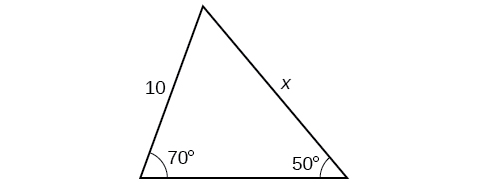$12.3$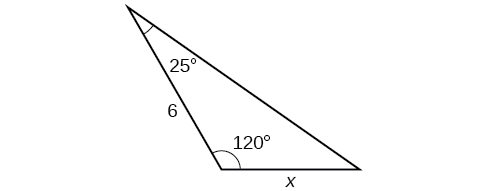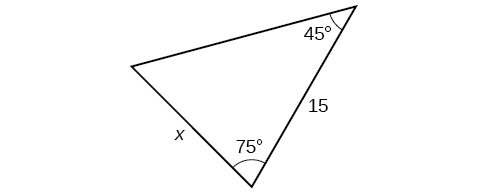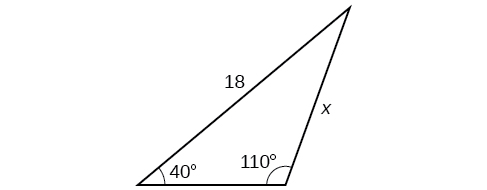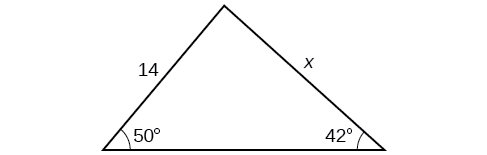For the following exercises, find the measure of angle $\text{\hspace{0.17em}}x,\text{\hspace{0.17em}}$ if possible. Round to the nearest tenth.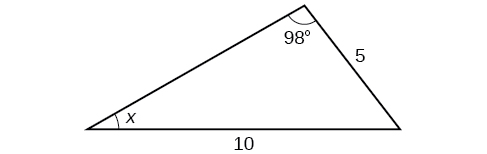$29.7°$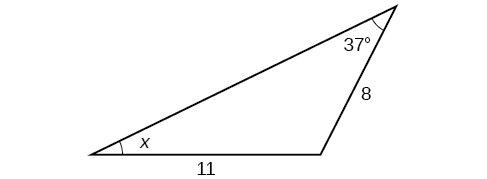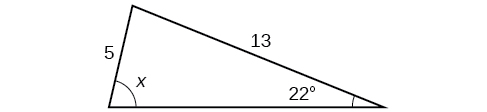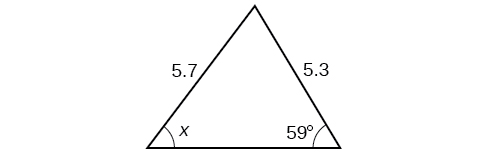Notice that $\text{\hspace{0.17em}}x\text{\hspace{0.17em}}$ is an obtuse angle.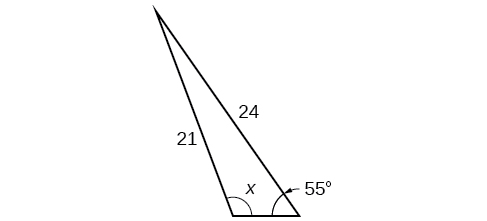$110.6°$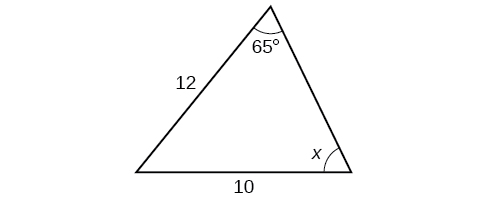For the following exercises, find the area of each triangle. Round each answer to the nearest tenth.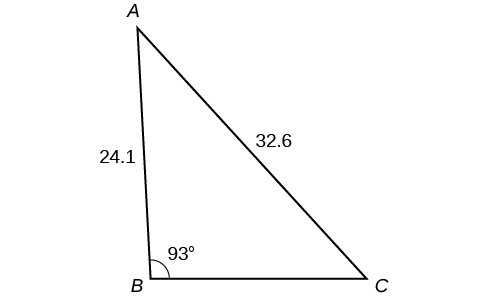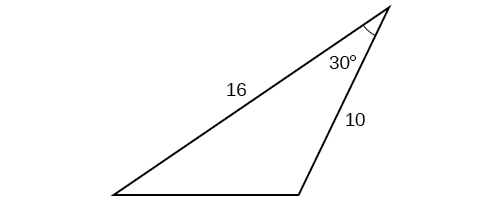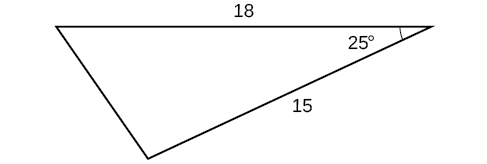$57.1$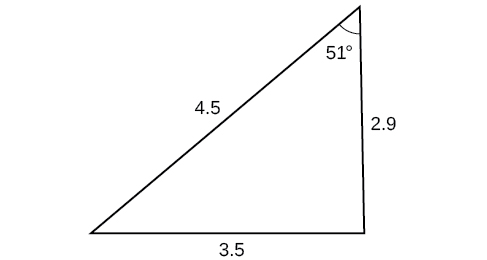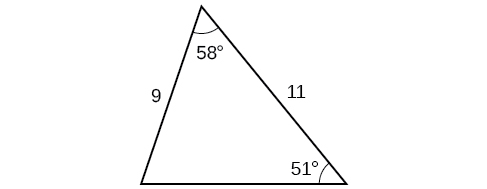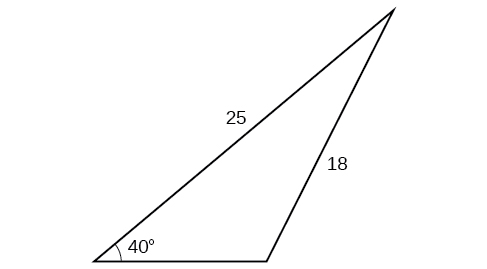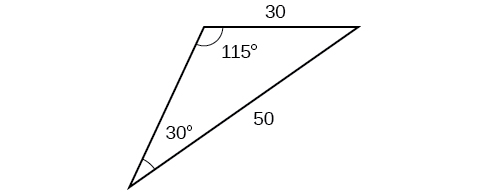## Extensions

Find the radius of the circle in [link] . Round to the nearest tenth.

Find the diameter of the circle in [link] . Round to the nearest tenth.

$10.1$

what are odd numbers
numbers that leave a remainder when divided by 2
Thorben
1,3,5,7,... 99,...867
Thorben
7%2=1, 679%2=1, 866245%2=1
Thorben
the third and the seventh terms of a G.P are 81 and 16, find the first and fifth terms.
if a=3, b =4 and c=5 find the six trigonometric value sin
pls how do I factorize x⁴+x³-7x²-x+6=0
in a function the input value is called
how do I test for values on the number line
if a=4 b=4 then a+b=
a+b+2ab
Kin
commulative principle
a+b= 4+4=8
Mimi
If a=4 and b=4 then we add the value of a and b i.e a+b=4+4=8.
Tariq
what are examples of natural number
an equation for the line that goes through the point (-1,12) and has a slope of 2,3
3y=-9x+25
Ishaq
show that the set of natural numberdoes not from agroup with addition or multiplication butit forms aseni group with respect toaaddition as well as multiplication
x^20+x^15+x^10+x^5/x^2+1
evaluate each algebraic expression. 2x+×_2 if ×=5
if the ratio of the root of ax+bx+c =0, show that (m+1)^2 ac =b^2m
By the definition, is such that 0!=1.why?
(1+cosA+IsinA)(1+cosB+isinB)/(cos@+isin@)(cos$+isin$)
hatdog
Mark
jaks
Ryan

#### Get Jobilize Job Search Mobile App in your pocket Now!ByByBy Anh DaoBy Saylor FoundationBy OpenStaxBy Brooke DelaneyBy Janet ForresterBy Sebastian Sieczko...By OpenStaxBy OpenStaxBy Brooke DelaneyBy Courntey Hub